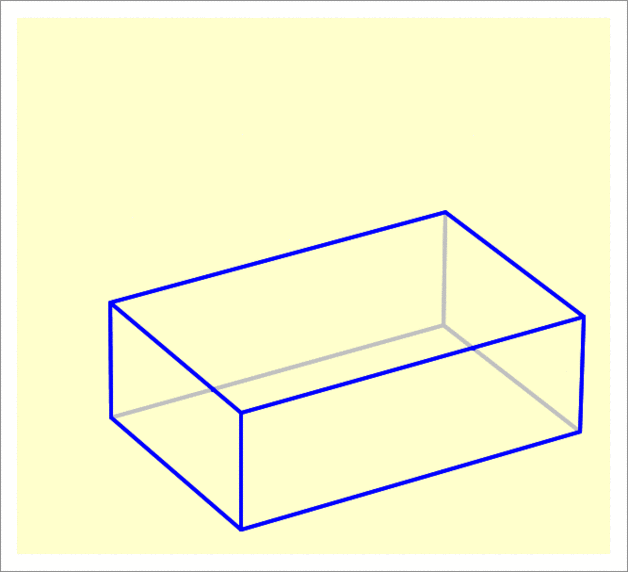# Rotate that Cuboid

Geometry Level 2

A cuboid has base dimensions $30 \times 20$ and a height of $10$ and is lying flat on the $xy$-plane.

It undergoes two rotations—the first is $30^{\circ}$ about the edge measuring $20$, and the second is also $30^{\circ}$ about the edge measuring $30$. Both rotations are such that the center of the cuboid is raised upward.

Find the $z$-coordinate (that is, the altitude) of the highest point of the cuboid after applying the two rotations (to 2 decimal places).×

Problem Loading...

Note Loading...

Set Loading...